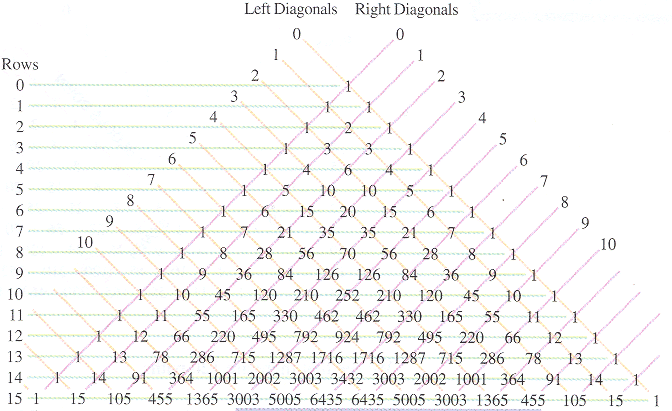# Section 12.2: Combinations

## 12.2 Outline

1. Committee problem
1. definition
2. combination formula
3. deck of cards
2. Pascal’s triangle
1. n choose r
2. table entries
3. Counting with the binomial theorem
1. binomial theorem
2. number of subsets

## 12.2 Essential Ideas

Combinations
A combination of r elements selected from a set of n elements is an subset of r elements selected without repetitions. The order of selection is not important.

Counting Formulas

Combination formula: The number of ways of selecting r elements from a set with cardinality n in which the order of selection is not important is n!/r!(n − r)!.

Combinations by Pascal’s Triangle
The number of ways of selecting r elements from a set with cardinality n in which the order of selection is not important is found by looking at the rth entry in the n row: